# ggpolar: Dots and Their Connections in Polar Coordinate System

`{ggpolar}` provides a very flexible way to create dots in coordinate system for event list and connect the dots with segments based on `{ggplot2}`.

## Installation

You can install the released version of `{ggpolar}` from CRAN with:

``install.packages("ggpolar")``

You can install the development version of `{ggpolar}` from GitHub with:

``remotes::install_github("ShixiangWang/polar")``

## Example

### Init a polar plot

``````library(ggpolar)

data <- data.frame(x = LETTERS[1:7])

p1 <- polar_init(data, x = x)
p1``````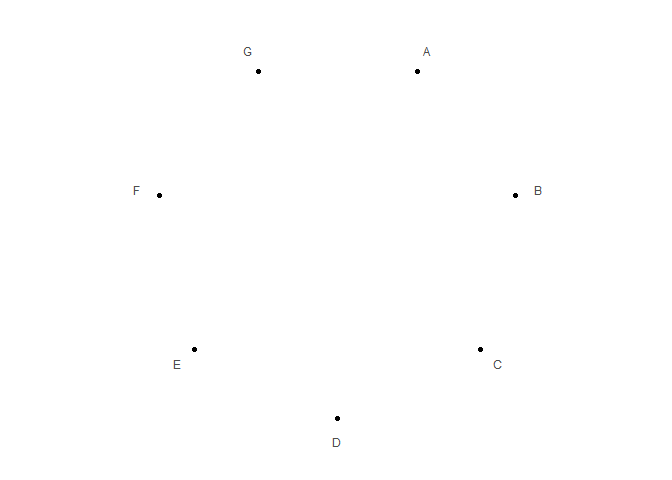``````# Set aes value
p2 <- polar_init(data, x = x, size = 3, color = "red", alpha = 0.5)
p2``````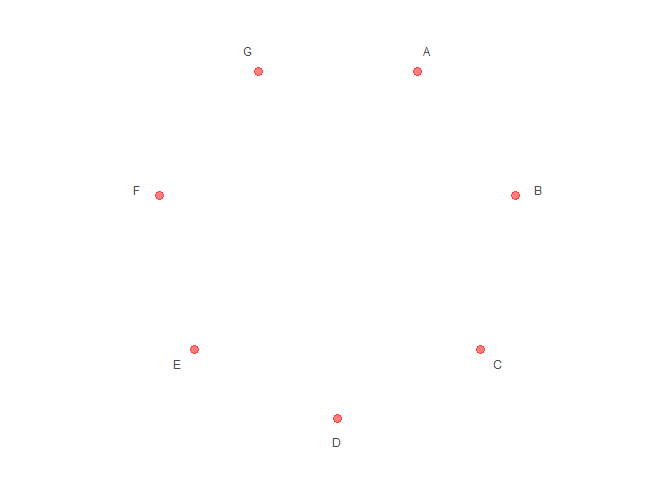``````# Set aes mapping
set.seed(123L)
data1 <- data.frame(
x = LETTERS[1:7],
shape = c("r", "r", "r", "b", "b", "b", "b"),
color = c("r", "r", "r", "b", "b", "b", "b"),
size = abs(rnorm(7))
)
# Check https://ggplot2.tidyverse.org/reference/geom_point.html
# for how to use both stroke and color
p3 <- polar_init(data1, x = x, aes(size = size, color = color, shape = shape), alpha = 0.5)
p3``````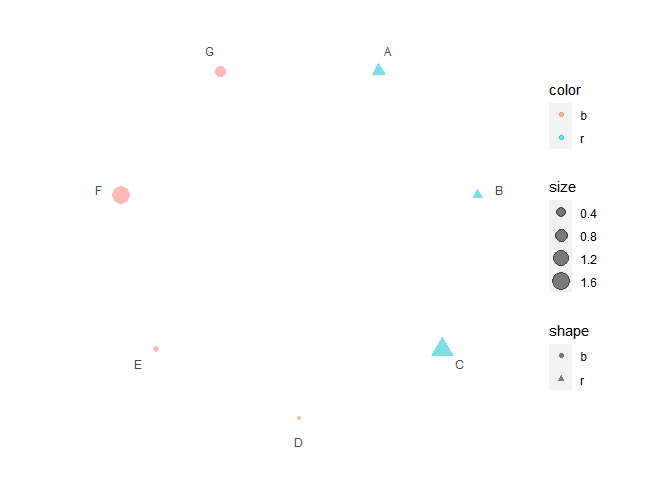### Connect polar dots

``````data2 <- data.frame(
x1 = LETTERS[1:7],
x2 = c("B", "C", "D", "E", "C", "A", "C"),
color = c("r", "r", "r", "b", "b", "b", "b")
)
p4 <- p3 + polar_connect(data2, x1, x2)
p4``````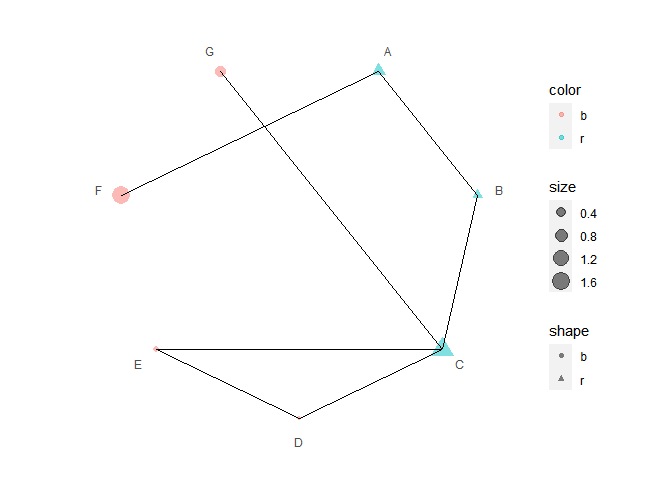``````# Unlike polar_init, mappings don't need to be included in aes()
p5 <- p3 + polar_connect(data2, x1, x2, color = color, alpha = 0.8, linetype = 2)
p5``````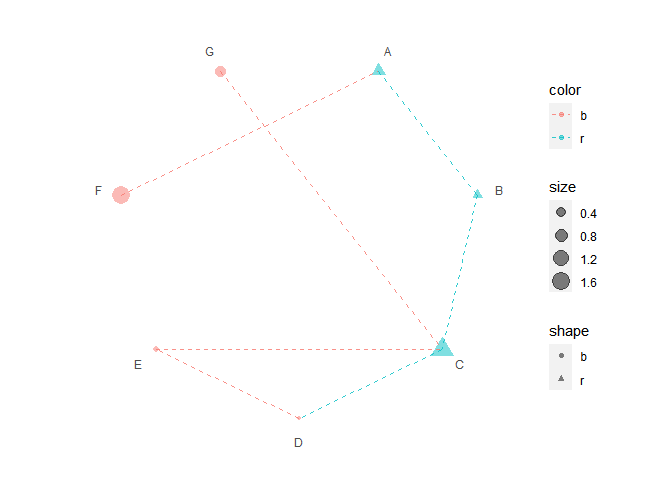``````# Use two different color scales
if (requireNamespace("ggnewscale")) {
library(ggnewscale)
p6 = p3 +
new_scale("color") +
polar_connect(data2, x1, x2, color = color, alpha = 0.8, linetype = 2)
print(p6 + scale_color_brewer())
print(p6 + scale_color_manual(values = c("darkgreen", "magenta")))
}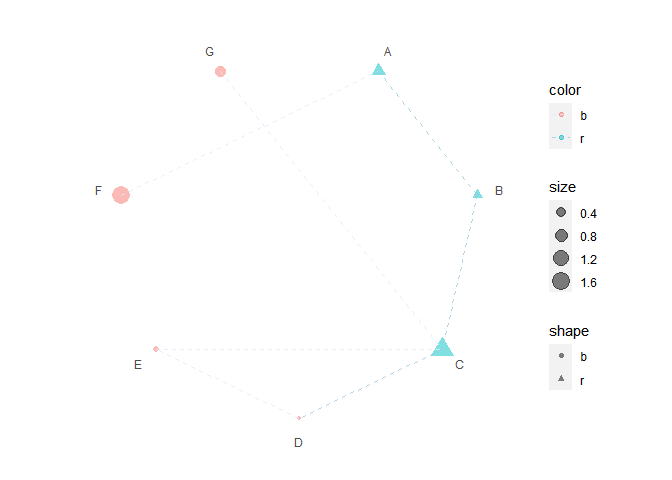If you use `{ggpolar}` in academic research, please cite the following paper along with the GitHub repo.